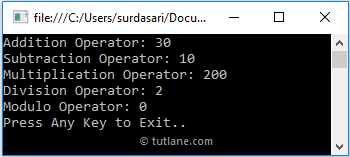# C# Arithmetic Operators with Examples

In c#, Arithmetic Operators are useful to perform basic arithmetic calculations like addition, subtraction, division, etc., based on our requirements.

For example, we have integer variables x = 20, y = 10, and if we apply an arithmetic operator + (x + y) to perform an addition operator. We will get the result as 30 like as shown below.

int result;
int x = 20, y = 10;
result = (x + y);

The following table lists the different types of operators available in c# arithmetic operators.

OperatorNameDescriptionExample (a = 6, b = 3)
- Subtraction It subtracts two operands. a - b = 3
* Multiplication It multiplies two operands. a * b = 18
/ Division It divides numerator by de-numerator. a / b = 2
% Modulo It returns a remainder as a result. a % b = 0

## C# Arithmetic Operators Example

Following is the example of using the Arithmetic Operators in the c# programming language.

using System;

namespace Tutlane
{
class Program
{
static void Main(string[] args)
{
int result;
int x = 20, y = 10;
result = (x + y);
result = (x - y);
Console.WriteLine("Subtraction Operator: " + result);
result = (x * y);
Console.WriteLine("Multiplication Operator: "+ result);
result = (x / y);
Console.WriteLine("Division Operator: " + result);
result = (x % y);
Console.WriteLine("Modulo Operator: " + result);
Console.WriteLine("Press Enter Key to Exit..");
}
}
}

If you observe the above code, we used Arithmetic operators (+, -, *, /, %) to perform different operations on defined operands based on our requirements.

## Output of C# Arithmetic Operators Example

When we execute the above c# program, we will get the result as shown below.This is how we can use arithmetic operators in the c# programming language to perform the basic arithmetic calculations on operands based on our requirements.